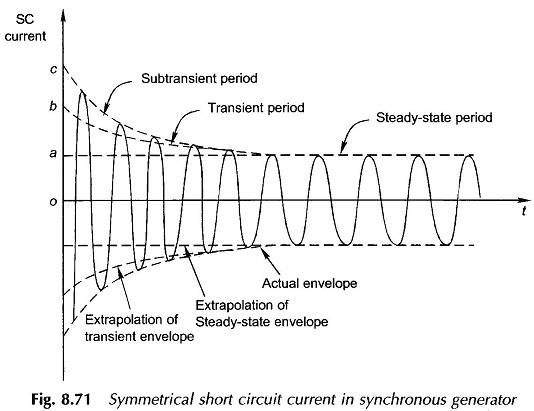# Iterative Method of solving multiple variables without using the curvefit tool

2 ビュー (過去 30 日間)
Harry Bumfrey 2019 年 7 月 26 日
コメント済み: Harry Bumfrey 2019 年 7 月 28 日
Hi everyone,
Any form of help would be greatly appreciated. :)
Background:
I am a placement student and have used MATLAB (and various tools inside) quite extensively throughout my placements and degree. However my statistical knowledge of error methods is quite low and is hindering me completing this task. At the moment I am using an iterative method to try and curvefit a waveform from a test environment.
Using-
Iterative_waveform(I,:) = ((Vq1/xd)+(((Vq1/xDd)-(Vq1/xd))*exp(-t1/TDd))+(((Vq1/xDDd)-(Vq1/xDd))*exp(-t1/TDDd))).*sin(2*pi*f1*t1);
(This is the short current waveform of a synchrounous machine)
Vq1 = 556
t1 = known
xd = unknown
xDd = unknown
TDd = unknown
TDDd = unknown
xDDd = unknownProblem:
1. The curvefit tool cannot curvefit the waveform even with the equation therefore I am trying to produce an iterative method to produce the correct variables
2. Using an r^2 value on a sine wave is inaccurate, due to the fact that a perfect waveform could be out of phase from the original data. I can't find an error checking method for accrurately producing a error.
Question:
1. does anyone know of a robust error checking method for a sinewave (was thinking of abs() then using r^2)
2. does know how to converge using an error method for a sine wave due to the r^2 values increasing and decreasing through all iterations

サインインしてコメントする。

### 回答 (1 件)

David Wilson 2019 年 7 月 26 日
Here's my attempt at fitting uusing lsqcurvefit from the optimisation toolbox.
First I generate some synthetic data to subsequently fit that follows your curve (since you didn't think to give us any)
Vq1 = 556; % known constant
N = 200; % # of points
t = linspace(0,0.2,N)';
f = 50; % presumably 50 Hz.
% Set values for constants
xd = 1;
xDd = 2;
TDd = 0.1;
TDDd = 0.05;
xDDd = 0.3;
phi = -0.6; % initial phase offset
y = (Vq1/xd+((Vq1/xDd-Vq1/xd))*exp(-t/TDd) + ...
(((Vq1/xDDd)-(Vq1/xDd))*exp(-t/TDDd))).* ...
sin(2*pi*f*t+phi);
plot(t,y,'.-')
Now we are ready to do the fitting:
%% Now use lsqcurvefit
p0 = 3*[1,1,1, 1, 1, 1]'; % guess of parameters
LB = [0,0,0, 0, 0, -5]'; % can put anything here, even -inf.
UB = 10*[1,1,1, 1, 1, 1]';
fun = @(p, t) (Vq1/p(1)+((Vq1/p(2)-Vq1/p(1)))*exp(-t/p(3)) + ...
(((Vq1/p(5))-(Vq1/p(2)))*exp(-t/p(4)))).* ...
sin(2*pi*f*t+p(6));
pfit = lsqcurvefit(fun,p0,...
t,y, ...
LB,UB)
yfit = fun(pfit,t);
plot(t,y,'r.', t,yfit,'b-');
We get a pretty good fit since I start reasonably near the values used to generate the original curve.#### 1 件のコメント

Harry Bumfrey 2019 年 7 月 28 日
Thanks, yeh sorry unfortuantly im unable to give physical data information due to company confidentiality.

サインインしてコメントする。

### Community Treasure Hunt

Find the treasures in MATLAB Central and discover how the community can help you!

Start Hunting!